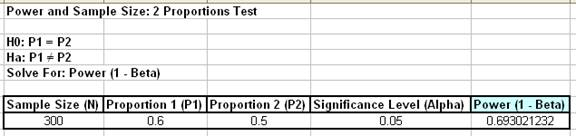Include Top

# How Do I Perform Power and Sample Size calculations for Two Proportions Test?

To determine Power & Sample Size for a 2 Proportions Test, you can use the Power & Sample Size Calculator or Power & Sample Size with Worksheet.

1. Click SigmaXL > Statistical Tools > Power & Sample Size Calculators > 2 Proportions Test Calculator.
2. Select Solve For Power (1 – Beta). Enter Sample Size and Proportion 1 values as shown:Note that we are calculating the power or likelihood of detection given that the maximum difference between group means = 1, with sample size for each group = 30, 3 groups, standard deviation = 1, significance level = .05, and Ha: Not Equal To (two sided test).

3. Click OK. The resulting report is displayed:

4.A power value of 0.69 is unacceptable. Note that this value is much less than the power for the one proportion test (0.94).

5. To compensate, we will double the sample size per group. Press F3 or click Recall SigmaXL Dialog to recall last dialog. Change the sample size per group from 300 to 600. Note that the power value is now 0.94.
6. To determine Power & Sample Size using a Worksheet, click SigmaXL > Statistical Tools > Power & Sample Size with Worksheet > 2 Proportions Test.
7. A graph showing the relationship between Power, Sample Size and Proportion Values can then be created using SigmaXL > Statistical Tools > Power & Sample Size Chart. See here for an example using the 1 Sample t-Test.

# Web Demos

Our CTO and Co-Founder, John Noguera, regularly hosts free Web Demos featuring SigmaXL and DiscoverSim